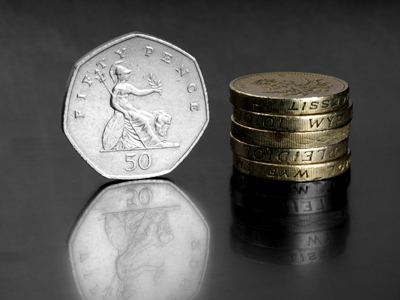How many 50p pieces would you need to make up £5?

# Year 2 Money - Value of Coins

This quiz addresses the requirements of the National Curriculum KS1 Maths and Numeracy for children aged 6 and 7 in year 2. Specifically this quiz is aimed at the section dealing with recognising coin values and finding different combinations of coins that equal the same amounts of money.

Children in Year 2 should be familiar with all the coins we use and their values. They should also recognise the values of different combinations, for example, that two £1 coins have the same value as a £2 coin or that two 50p coins have the same value as a £1 coin.

This quiz will help children to work out the values of different coin combinations.

To see a larger image, click on the picture.
Question 1
If I had 5 of these coins, I would have the same value as a...
20p piece
£1 coin
50p piece
£2 coin
Five ten pence pieces has the same value as one 50p piece
Question 2
What is the value of one of these coins?
2p
1p
5p
10p
A 2p coin has the same value as two 1p coins
Question 3
This coin is worth the same as...
two 50p pieces
two £1 coins
ten 5p pieces
six 2p pieces
This is a £2 coin, worth 2 x £1
Question 4
How many of these coins would have the same value as £1?
100
10
50
20
There are 100 pennies in a pound
Question 5
Which coin here has the lowest value?
1p
2p
10p
They all have the same value
The 1p is the lowest value coin we use
Question 6
Together these coins have the same value as...
10p
20p
£1
£2
2 x 50p = 100p and 100p = £1
Question 7
What is the value of one of these coins?
£1
20p
10p
5p
5p coins are much smaller than 20p and 10p pieces
Question 8
Which coin is missing?
£1
£2
50p
2p
We have 8 different coins altogether
Question 9
How many 2p coins would have the same value as 20p?
20
10
2
5
10 x 2 = 20
Question 10
What is the highest value coin in this handful?
£1
50p
20p
£2
The £2 coin is the highest value coin we have
You can find more about this topic by visiting BBC Bitesize - How to work out a value with coins

Author:  Angela Smith